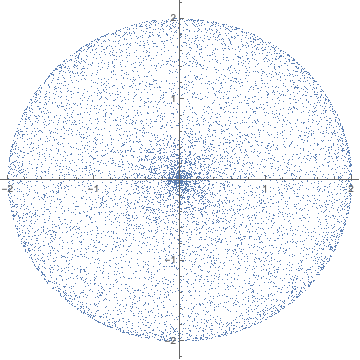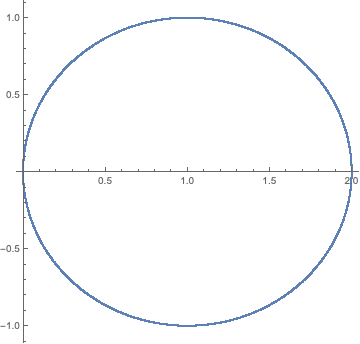# Is the random point $(C,S)$ the same as $(1,0)+(\cos U,\sin U)=(1 + \cos U,\sin U)$, with $U$ a uniform r.v.?

Suppose that $$\theta_1$$ and $$\theta_2$$ are independent and identically distributed (i.i.d.) random variables and that $$\theta_j$$ has probability density function (PDF) $$f_j = \frac{1}{2\pi}$$ ($$i.e.$$, the uniform distribution) for $$j = 1$$ and $$2$$. Next, we define the following random variables $$C = \cos \theta_1 + \cos \theta_2$$ and $$S = \sin \theta_1 + \sin \theta_2$$.

My question is, can I say, based on the rotational invariance, that the random point $$(C,S)$$ is the same as that of $$(1,0)+(\cos U,\sin U)=(1 + \cos U,\sin U)$$, where $$U$$ is uniformly distributed on $$[0,2\pi]$$? If so, how can I show that?

These two scatter plots illustrate the difference, the first is for the points $$(C,S)=(\cos\theta_1+\cos\theta_2,\sin\theta_1+\sin\theta_2)$$, the second for the points $$(1+\cos U,\sin U)=(1+\cos\theta_3,\sin\theta_3)$$, where all angles $$\theta_i$$ are uniformly distributed in $$(0,2\pi)$$.The second distribution uniformly fills a unit circle with center at $$(1,0)$$. The first distribution fills a disc with radius 2, but not uniformly, the density diverges as $$1/r$$ at a distance $$r$$ from the origin.

To calculate the radial distribution $$P(r)$$, it is helpful to think of a random walk on the plane with unit step size and random orientation. The desired $$P(r)$$ is the distribution of the distance from the origin after two steps.

After the first step the random walker is at some arbitrary point on the unit circle. For the distribution $$P(r)$$ it does not matter where on the unit circle, we may place the point at $$(1,0)$$. Then the second step brings the random walker to the point $$(1+\cos\phi,\sin\phi)$$, with $$\phi$$ uniformly in $$(0,2\pi)$$. The distance from the origin is $$r=\sqrt{2+2\cos\phi}$$, and the probability distribution is $$P(r)=\frac{2/\pi}{\sqrt{4-r^2}},\;\;0 The corresponding density $$\rho(r)=P(r)/2\pi r$$ indeed diverges $$\propto 1/r$$ when $$r\rightarrow 0$$.

For the generalization to $$(C_n,S_n)=(\sum_{i=1}^n\cos\theta_i,\sum_{i=1}^n\sin\theta_i)$$ see this MO posting.

• Dear, thanks for you answer. I have found the same conclusions and for me they are not the same distribution, however, this answer: mathoverflow.net/questions/347211/… states that they are the same and the derived PDF matches the simulations. – Felipe Augusto de Figueiredo Nov 30 '19 at 21:35
• I have explained what the relationship is between the two plots: the plot on the right has the same probability distribution of the distance from the origin as the plot on the left. I'm sure that is what is meant in the linked answer, not that the plots themselves are the same. – Carlo Beenakker Nov 30 '19 at 21:49